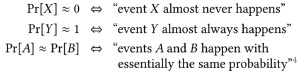4.2: Negligible Probabilities

In all of the cryptography that we’ll see, an adversary can always violate security simply by guessing some value that was chosen at random, like a secret key. However, imagine a system that has 1024-bit secret keys chosen uniformly at random. The probability of correctly guessing the key is 2−1024, which is so low that we can safely ignore it.

We don’t worry about “attacks” that have such a ridiculously small success probability. But we would worry about an attack that succeeds with, say, probability 1/2. Somewhere between 2−1024 and 2−1 we need to find a sensible place to draw the line. In the same way that polynomial time formalizes “efficient” running times, we will use an asymptotic definition of what is a negligibly small probability.

Consider a scheme with keys that are λ bits long. Then a blind guess is correct with probability 1/2λ. Now what about an adversary who makes 2 guesses, or λ guesses, or λ42 guesses? Such an adversary would still run in polynomial time, and might succeed in its attack with probability 2/2λ , λ/2λ , or λ42/2λ.

Our starting point here is 1/2λ, and the nice thing about that probability is that, no matter what polynomial we place on top, we still get a very small probability indeed. This idea gives rise to our formal definition:

Definition $$\PageIndex{1}$$: Negligible

A function ? is negligible if, for every polynomial p, we have limλ→∞ p(λ)?(λ) = 0.

In other words, a negligible function approaches zero so fast that you can never catch up when multiplying by a polynomial. This is exactly the property we want from a security guarantee that is supposed to hold against all polynomial-time adversaries. If a polynomial-time adversary succeeds with probability ?, then running the same attack ptimes would still be an overall polynomial-time attack (if p is a polynomial), and potentially have success probability p · ?.

Example $$\PageIndex{1}$$:

The function ?(λ) = 1/2λ is negligible, since for any polynomial p(λ) = λc, we have:since clog(λ) − λ approaches −∞ in the limit, for any constant c.

Similarly, functions like 1/2λ/2, 1/2log2 λ, and 1/nlog n are all negligible.

In this class, when we see a negligible function, it will typically always be one that is easy to recognize as negligible (just as in an undergraduate algorithms course, you won’t really encounter algorithms where it’s hard to tell whether the running time is polynomial).

Definition $$\PageIndex{2}$$: (f ≈ g)

If ?,g : ℕ → ℝ are two functions, we write ? ≈ g to mean that |?(λ) − g(λ)| is a negligible function.

We use the terminology of negligible functions exclusively when discussing probabilities, so the following are common:Additionally, the ≈ symbol is transitive:5 if Pr[X] ≈ Pr[Y] and Pr[Y] ≈ Pr[Z], then Pr[X] ≈ Pr[Z] (perhaps with a slightly larger, but still negligible, difference).

4Pr[A] ≈ Pr[B] doesn’t mean that events A and B almost always happen together (when A and B are defined over a common probability space) — imagine A being the event “the coin came up heads” and B being the event “the coin came up tails.” These events have the same probability but never happen together. To say that “A and B almost always happen together,” you’d have to say something like Pr[AB] ≈ 0, where AB denotes the event that exactly one of A and B happens.

5It’s only transitive when applied a polynomial number of times. So you can’t define a whole series of events Xi, show that Pr[Xi] ≈ Pr[Xi+1], and conclude that Pr[X1] ≈ Pr[X2n]. It’s rare that we’ll encounter this subtlety in this course.